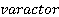Create an Account

Home / Questions / A silicon diode exhibits a capacitance of 200 pF at zero bias If it is in parallel with ...

A silicon diode exhibits a capacitance of 200 pF at zero bias If it is in parallel with a 60 pF capacitor and 200 µH inductor calculate the range of resonant frequency as the diode vaiies

. A silicondiode exhibits a capacitance of 200 pF at zero bias. If it is in parallel with a 60-pF capacitor and 200-µ,H inductor, calculate the range of resonant frequency as the diode vaiies through a reverse bias of 3- 15 V. (966 kHz, 1.15 MHz)

2. Adiode has C0 equal to 320 pF. Plot a curve of capacitance versus VR from 0 to 20 V. The diode is used with a 200-µ,H coil. Plot the resonant frequency versus V R from 0 to 20 V and suggest how the response could be linearized.

Jul 29 2020 View more View LessSubscribe To Get Solution